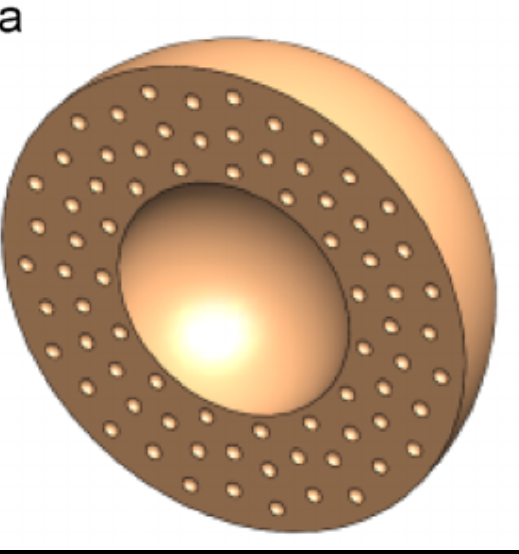# Is it possible to generate pores in surface?

Hi want to generate 3d pores in the surface of sphere something like that.Please suggest me how it can be done in Mathematica.

There are some nice tools using Derived Geometric Regions that you can use for this

For example:

router = 2; (*outside radius*)
rsmall = 0.1; (*radius of small pores*)
nsmall = 40; (*number of small pores*)
radii = RandomReal[{rinner + 2*rsmall, router - 2 rsmall},nsmall];(*radial position of smallpores*)

angle1 = RandomReal[{0, 2 $\Pi$},nsmall];(*angular position of small pores*)
coords = Map[radii[[#]]*{0, Sin[angle1[[#]]], Cos[angle1[[#]]]} &,Range[nsmall]];(*coordinates of small pores*)
hollowsphere = RegionDifference[Ball[{0, 0, 0}, 2], Ball[{0, 0, 0}, 1]];(*first region interesection*)
smallspheres =  RegionUnion[Map[Ball[coords[[#]], rsmall] &, Range[nsmall]]];(*combine small pores*)

hollowsphereminussmallspheres = RegionDifference[hollowsphere, smallspheres] (*second region interesection*)
r1 = ImplicitRegion[x < 0, {x, y, z}]; (*For visualization*)
rplot = RegionIntersection[r1, hollowsphereminussmallspheres];


Then use this to plot:

RegionPlot3D[rplot, PlotPoints -> 100]


giving:Not exactly as the image but if you play with the arrangement of the pores you should get what you want.

• You might be interested in SphericalShell[]. If non-overlapping pores are wanted, one could adapt the strategies in this thread to this case. – J. M. will be back soon Mar 24 '17 at 8:07